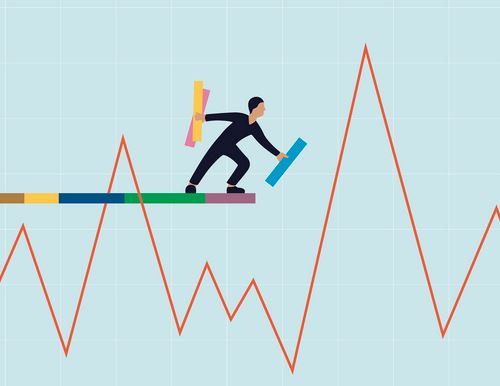## How to Deal With Market Volatility in Your Favour?

Jan 24 • Forex Trading Articles • 292 Views • Comments Off on How to Deal With Market Volatility in Your Favour?

You’ll inevitably encounter volatility no matter what market you choose. It’s a vital aspect of a financial market. The most common mistake new investors make is believing volatility will appear and disappear over time.

Let’s clarify how to exploit the power of price volatility for your investment success. Volatility always exists. The only difference is its strength.

## What is volatility?

This term refers to determining market fluctuations and whether an asset is risky. Volatility can be determined using standard deviation, a measure that measures the rate at which prices change for a set of returns.

There are different degrees of volatility: high, medium, and low. Higher volatility translates to more risk. Volatility determines market fluctuation and determines whether an asset is risky.

For example, the oil market is renowned for its high volatility, while the silver market has low volatility. The volatility of these markets and securities is well known. As far as forex is concerned, the Australian and New Zealand dollar are both highly volatile. The degree of volatility, however, will depend on the timeframe used.

## How to calculate volatility?

Two main methods exist to calculate volatility: variance and standard deviation. Using the following steps, you can see how they work together:

• Consider counting volatility for the past ten days. To do that, you need the close prices for those days. Excel makes calculating volatility easier.
• Next, add each number and divide it by the number of values to find the average.
• Suppose that the close prices increase from \$10 to \$100. Consequently, our first day has \$10; the second has \$20, and so on. This gives us \$550. Together, we divide 550 by 10 to get \$55.
• In the third step, you need to find the deviation by subtracting the average from each figure. For example, \$100 minus \$75 = \$45, \$ 90 plus \$75 = \$35, etc. You are likely to have negative averages in this method.
• Squaring the deviations will remove the negative values.
• The total squared deviations add up to 825.
• In the next step, we will divide the previous sum by the number of figures (10), which results in \$82.50.
• This number shows how far the price can deviate from the average when calculated using the square root of \$82.50.

## Tips for trading in volatile markets

Following are some tips to consider when trading during volatile markets:

• You need to determine which type of volatility suits your trading strategy. Should you take a risk by trading during high volatility, or should you avoid it?
• There are three common indicators to gauge volatility, so choose the most relevant to your strategy. Having assistants will help you determine how volatile a market is.
• Invest in your volatility strategy. There is no perfect volatility strategy. If you want to trade on high volatility, make sure that you check the economic calendar or news to find out what events may cause markets to become extremely volatile. Use indicators to help you avoid market volatility if you prefer to avoid it.

## Bottom line

The volatility of any market is an integral part of the whole process. To be successful in investing or trading, one must understand what factors influence the volatility level. High volatility is better than many think since it yields rapid results. Furthermore, trading with a reliable broker will help you avoid many obstacles.